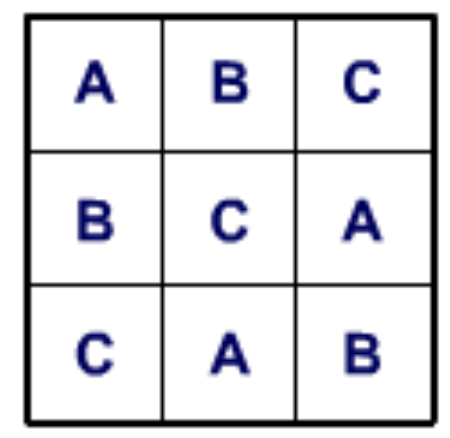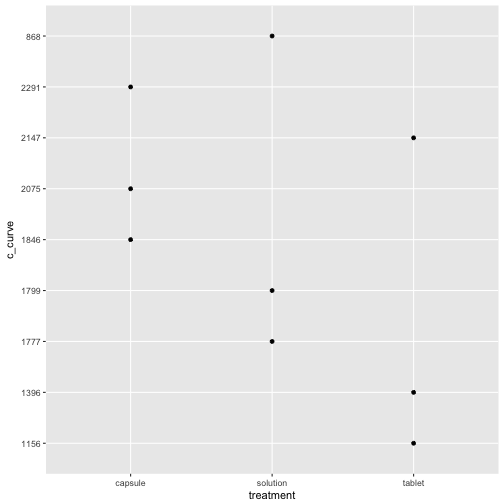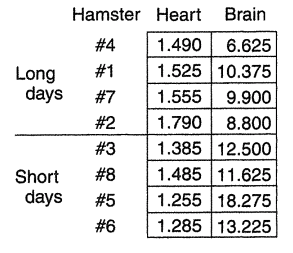# Split Plot/Repeated Measures Design

Prof Randi Garcia
April 2, 2018

1. There are two types of error in a split plot design. Explain the kids on boats analogy from page 277. Which type of error is represented by what movement in the analogy?
2. How do you compute the F ratio for the between-blocks factor? What about the within-blocks factor?

### Announcements

• Craig Krebsbach, Lab Instructor candidate, talk/demo today 12:15-1:15p in Bass 002
• Pizza will be served!
• HW 7 is due on Wed
• Project Method draft due today at Midnight on Moodle

### Agenda

• Latin square designs
• Split plot designs

### Inappropriate Insects

Modern zoos try to reproduce natural habitats in their exhibits as much as possible. They try to use appropriate plants, but these plants can be infested with inappropriate insects. Cycads (plants that look vaguely like palms) can be infected with mealybugs, and the zoo wishes to test three treatments: 1) water, 2) horticultural oil, and 3) fungal spores in water. Five infested cycads are taken to the testing area. Three branches are randomly selected from each tree, and 3 cm by 3 cm patches are marked on each branch. The number of mealybugs on the patch is counted. The three treatments then get randomly assigned to the three branches for each tree. After three days the mealybugs are counted again. The change in number of mealybugs is computed ($$before-after$$).

### Formal ANOVA for CB

${y}_{ij}={\mu}+{\tau}_{i}+{\beta}_{j}+{e}_{ij}$

Source SS df MS F
Treatment $$\sum_{i=1}^{a}b(\bar{y}_{i.}-\bar{y}_{..})^{2}$$ $$a-1$$ $$\frac{{SS}_{T}}{{df}_{T}}$$ $$\frac{{MS}_{T}}{{MS}_{E}}$$
Blocks $$\sum_{j=1}^{b}a(\bar{y}_{.j}-\bar{y}_{..})^{2}$$ $$b-1$$ $$\frac{{SS}_{B}}{{df}_{B}}$$ $$\frac{{MS}_{B}}{{MS}_{E}}$$
Error $$\sum_{i=1}^{a}\sum_{j=1}^{b}({y}_{ij}-\bar{y}_{i.}-\bar{y}_{.j}+\bar{y}_{..})^{2}$$ $$(a-1)(b-1)$$ $$\frac{{SS}_{E}}{{df}_{E}}$$

### Bioequivalence of drug delivery

This experiment is interested in the blood concentration of a drug after it has been administered. The concentration will start at zero, then go up, and back down as it is metabolized. This curve may differ depending on the form of the drug (a solution, a tablet, or a capsule). We will use three subjects, and each subject will be given the drug three times, once for each method. The area under the time-concentration curve is recorded for each subject after each method of drug delivery.

### Latin Square Design

In the bioequivalence example, because the body may adapt to the drug in some way, each drug will be used once in the first period, once in the second period, and once in the third period.

• We can use a Latin Square design to control the order of drug administration
• In this way, time is a second blocking factor### Latin Square Design

Treatments:

• Solution is treatment A
• Tablet is treatment B
• Capsule is treatment C
period 1 2 3
1 A 1799 C 2075 B 1396
2 C 1846 B 1156 A 868
3 B 2147 A 1777 C 2291

Factor diagram for the Latin Square??

### Latin Square Design

The actual data structure for analysis is “long.”

subject treatment period group c_curve
1 solution 1 A 1799
1 capsule 2 C 1846
1 tablet 3 B 2147
2 capsule 1 C 2075
2 tablet 2 B 1156
2 solution 3 A 1777
3 tablet 1 B 1396
3 solution 2 A 868
3 capsule 3 C 2291

### Informal ANOVA for Latin Square

We can make a parallel dot graphAnd check for equal standard deviations

library(mosaic)

sd <- favstats(c_curve ~ treatment, data = bioequivalence)[,8]

max(sd)/min(sd)

 2.387418


### Formal ANOVA for the Latin Square

${y}_{ijk}={\mu}+{\alpha}_{i}+{\beta}_{j}+{\tau}_{k}+{e}_{ijk}$

• $${\mu}$$ is the benchmark
• $${\alpha}_{i}$$ is the row effect
• $${\beta}_{j}$$ is the column effect
• $${\tau}_{k}$$ is the treatment effect
• There are p rows, columns, and treatments
Source SS df MS F
rows $$\sum_{i=1}^{p}p(\bar{y}_{i..}-\bar{y}_{...})^{2}$$ $$p-1$$ $$\frac{{SS}_{A}}{{df}_{A}}$$ $$\frac{{MS}_{A}}{{MS}_{E}}$$
columns $$\sum_{j=1}^{p}p(\bar{y}_{.j.}-\bar{y}_{...})^{2}$$ $$p-1$$ $$\frac{{SS}_{B}}{{df}_{B}}$$ $$\frac{{MS}_{B}}{{MS}_{E}}$$
treatment $$\sum_{k=1}^{p}p(\bar{y}_{..k}-\bar{y}_{...})^{2}$$ $$p-1$$ $$\frac{{SS}_{T}}{{df}_{T}}$$ $$\frac{{MS}_{T}}{{MS}_{E}}$$
Error $$\sum_{i=1}^{p}\sum_{j=1}^{p}({y}_{ijk}-\bar{y}_{i..}-\bar{y}_{.j.}-\bar{y}_{..k}+2\bar{y}_{..})^{2}$$ $$(p-1)(p-2)$$ $$\frac{{SS}_{E}}{{df}_{E}}$$

### Formal ANOVA for the Latin Square

ls_mod <- lm(c_curve ~ treatment + period + subject, data = bioequivalence)

anova(ls_mod)

Analysis of Variance Table

Response: c_curve
Df Sum Sq Mean Sq F value   Pr(>F)
treatment  2 608891  304445  67.733 0.014549 *
period     2 928006  464003 103.231 0.009594 **
subject    2 261115  130557  29.047 0.033282 *
Residuals  2   8990    4495
---
Signif. codes:  0 '***' 0.001 '**' 0.01 '*' 0.05 '.' 0.1 ' ' 1


### Split Plot/Repeated Measures Design